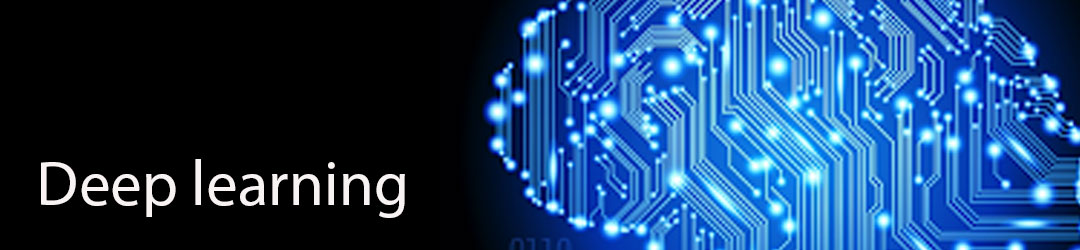+14107012688, +1 7327345775 training@techsmartits.com
Select Page# Deep Learning

Duration: 30 Hours

Part 1 – Artificial Neural Networks

All below sections will be implemented with tensorflow and keras, Programming knowledge of tensorflow and keras will be given during model buildings.

Section 1

1. Artificial Neural networks Intuition
2. Plan of Attack
3. The Neuron
4. The Activation Function
5. How do Neural Networks work?
6. How do Neural Networks learn?
9. Backpropagation

Section 2

1. Building an ANN
2. Prerequisites
3. How to get the dataset
5. Building an ANN – Step 1
6. Building an ANN – Step 2
7. Building an ANN – Step 3
8. Building an ANN – Step 4
9. Building an ANN – Step 5
10. Building an ANN – Step 6
11. Building an ANN – Step 7
12. Building an ANN – Step 8
13. Building an ANN – Step 9
14. Building an ANN – Step 10

Section 3

1. Homework Challenge – Should we say goodbye to that customer?
2. Homework Instruction
3. Homework Solution

Section 4

1. Evaluating, Improving and Tuning the ANN
2. Evaluating the ANN
3. Improving the ANN
4. Tuning the ANN

Part 2 – Convolutional Neural Networks

Section 5

1. CNN Intuition
2. What you’ll Need for CNN
3. Plan of attack
4. What are convolutional neural networks?
5. Step 1 – Convolution Operation
6. Step 1(b) – ReLU Layer
7. Step 2 – Pooling
8. Step 3 – Flattening
9. Step 4 – Full Connection
10. Summary
11. Softmax
12. Cross-Entropy

Section 6

1. Building a CNN
2. How to get the dataset
3. Introduction to CNNs
4. Building a CNN – Step 1
5. Building a CNN – Step 2
6. Building a CNN – Step 3
7. Building a CNN – Step 4
8. Building a CNN – Step 5
9. Building a CNN – Step 6
10. Building a CNN – Step 7
11. Building a CNN – Step 8
12. Building a CNN – Step 9
13. Building a CNN – Step 10

Section 7

1. Homework – What’s that pet?
2. Homework Instruction
3. Homework Solution
4. Evaluating, Improving and Tuning the CNN

Part 3 – Recurrent Neural Networks

Section 8

1. RNN (Recurrent Neural networks) Intuition
2. What you’ll need for RNN
3. Plan of attack
4. The idea behind Recurrent Neural Networks
6. LSTMs
7. Practical intuition
8. LSTM Variations

Section 9

1. Building a RNN
2. How to get the dataset
3. Building a RNN – Step 1
4. Building a RNN – Step 2
5. Building a RNN – Step 3
6. Building a RNN – Step 4
7. Building a RNN – Step 5
8. Building a RNN – Step 6
9. Building a RNN – Step 7
10. Building a RNN – Step 8
11. Building a RNN – Step 9
12. Building a RNN – Step 10
13. Building a RNN – Step 11
14. Building a RNN – Step 12
15. Building a RNN – Step 13
16. Building a RNN – Step 14
17. Building a RNN – Step 15

Section 10

1. Evaluating, Improving and Tuning the RNN
2. Evaluating the RNN
3. Improving the RNN
4. Tuning the RNN

Part 4 – Self Organizing Maps

Section 11

1. SOMs [Self-Organizing Maps] Intuition
2. Plan of attack
3. How do Self-Organizing Maps Work?
4. Why revisit K-Means?
5. K-Means Clustering (Refresher)
6. How do Self-Organizing Maps Learn? (Part 1)
7. How do Self-Organizing Maps Learn? (Part 2)
8. Live SOM example